# Resistance The Opposition to Electric Current Resistance Is

• Slides: 11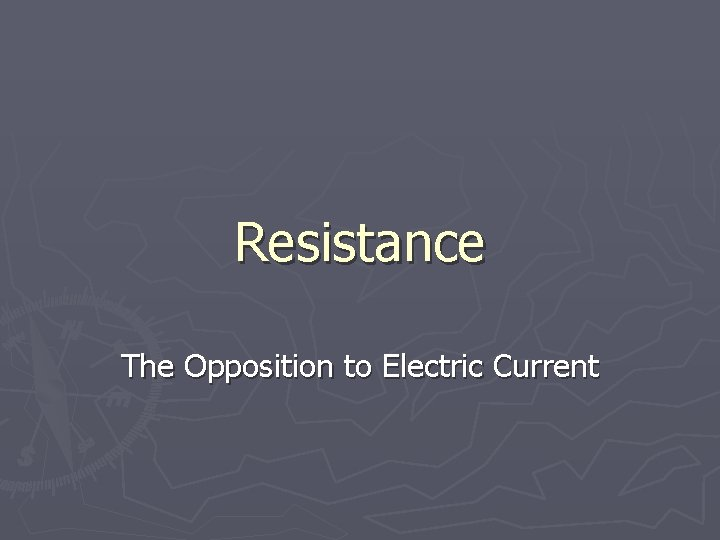Resistance The Opposition to Electric CurrentResistance ► Is the property of a substance that hinders a motion and converts electrical energy to other forms of energy, like heat, light & sound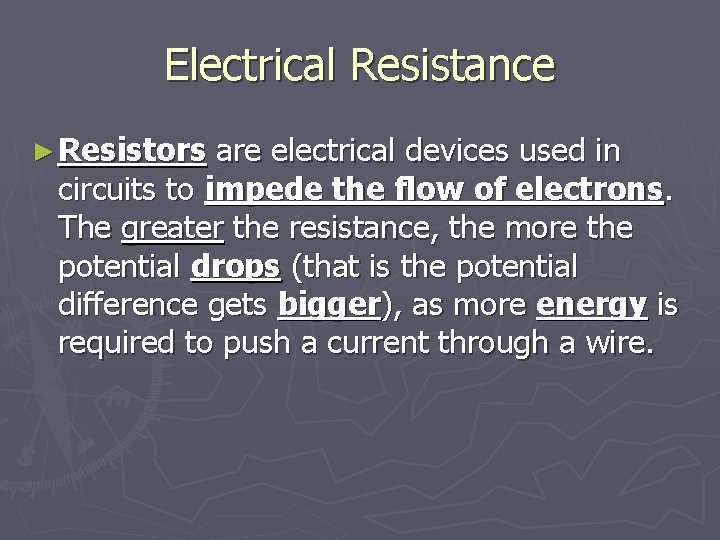Electrical Resistance ► Resistors are electrical devices used in circuits to impede the flow of electrons. The greater the resistance, the more the potential drops (that is the potential difference gets bigger), as more energy is required to push a current through a wire.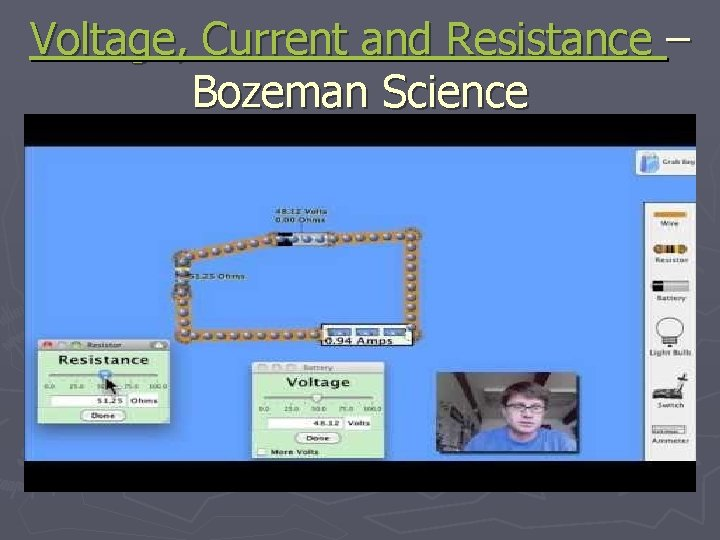Voltage, Current and Resistance – Bozeman Science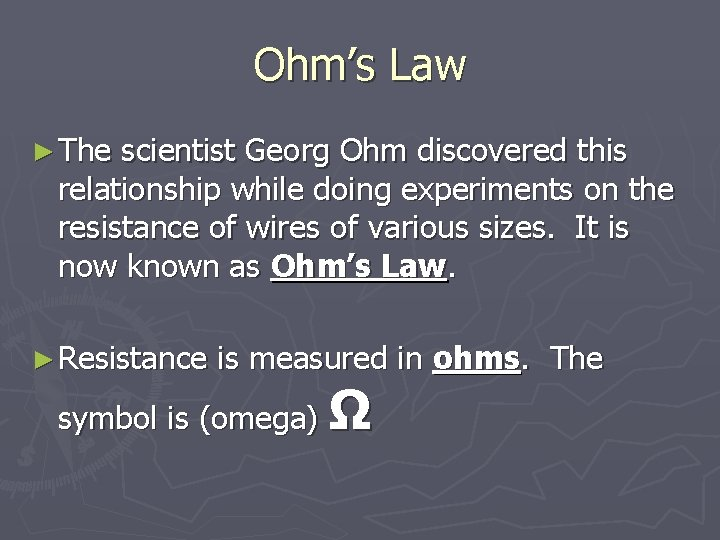Ohm’s Law ► The scientist Georg Ohm discovered this relationship while doing experiments on the resistance of wires of various sizes. It is now known as Ohm’s Law. ► Resistance is measured in ohms. The symbol is (omega) Ω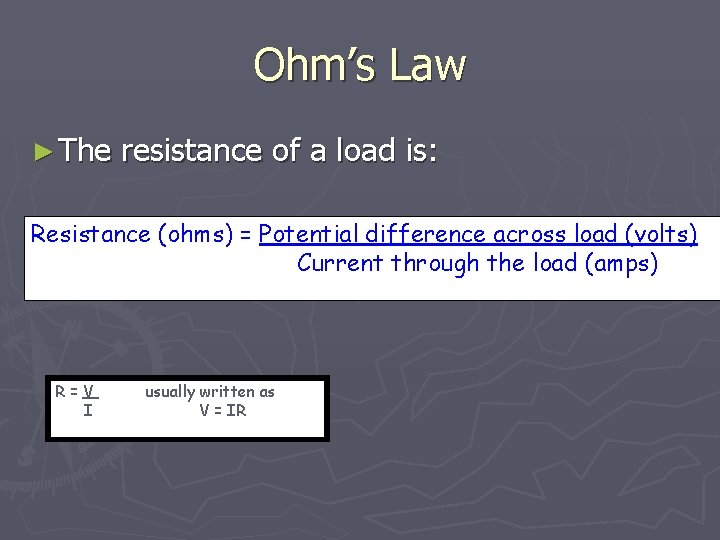Ohm’s Law ► The resistance of a load is: Resistance (ohms) = Potential difference across load (volts) Current through the load (amps) R=V I usually written as V = IR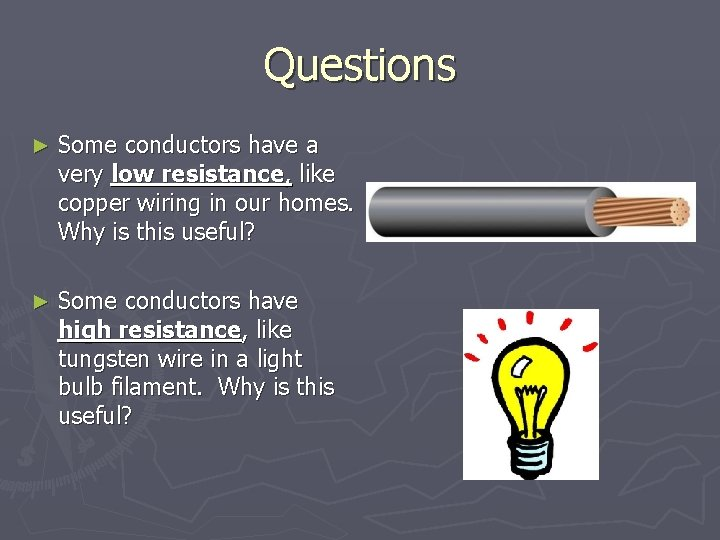Questions ► Some conductors have a very low resistance, like copper wiring in our homes. Why is this useful? ► Some conductors have high resistance, like tungsten wire in a light bulb filament. Why is this useful?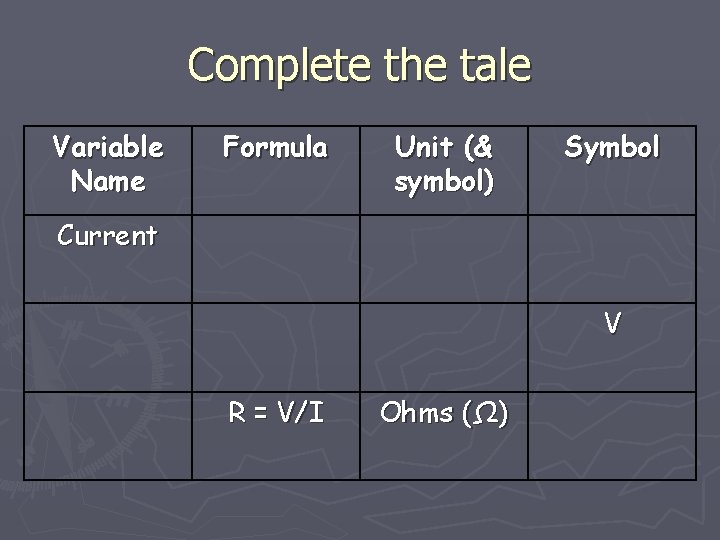Complete the tale Variable Name Formula Unit (& symbol) Symbol Current V R = V/I Ohms (Ω)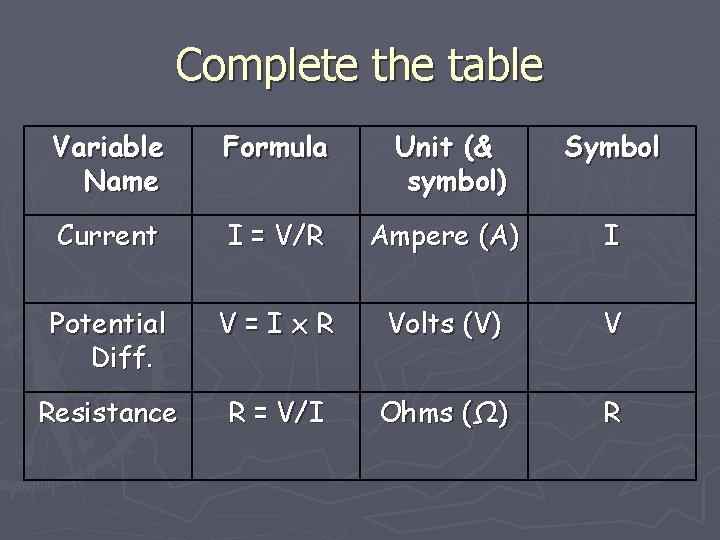Complete the table Variable Name Formula Unit (& symbol) Symbol Current I = V/R Ampere (A) I Potential Diff. V=Ix. R Volts (V) V Resistance R = V/I Ohms (Ω) RTVO Video ► Resistance: ► http: //www. youtube. com/watch? v=YGvu 9 iq j. Jq 4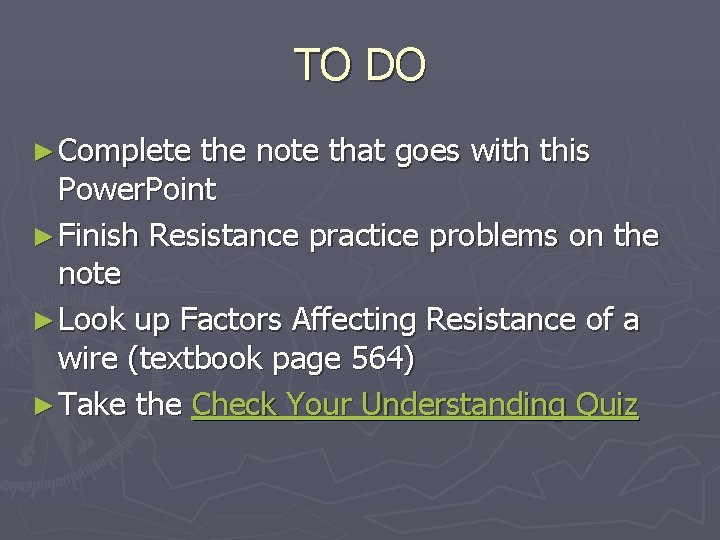TO DO ► Complete the note that goes with this Power. Point ► Finish Resistance practice problems on the note ► Look up Factors Affecting Resistance of a wire (textbook page 564) ► Take the Check Your Understanding Quiz